# Algebra 1 : Decimals and Percentage

## Example Questions

### Example Question #31 : Decimals And Percentage

Rewrite the following decimal as a percent.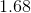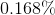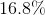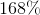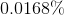Explanation:

Rewrite the following decimal as a percent.To convert between decimals and percents, we simply need to move the decimal point.

When going from a decimal to a percent, move the point two spaces to the right.

When going from a percent to a decimal, move the point two spaces to the left.

So this,Becomes### Example Question #41 : Decimals And Percentage

Convert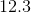to a percentage.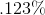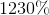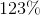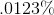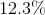Explanation:

To convert a decimal (or whole number) to a percentage, take the decimal and multiply it by 100.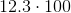### Example Question #2971 : Algebra 1

Convert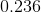to a percentage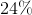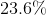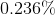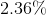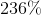Explanation:

To convert any decimal to a percentage, take the number and multiply it by 100. This is the same concept as moving the decimal point two places to the right.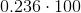This is all you need to do to convert it.

### Example Question #2971 : Algebra 1

Express the decimal as a percent.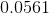5.61%

56.1%

0.000561%

0.561%

5.61%

Explanation:

To find the percent, we take the decimal and multiply it by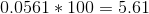The answer is then the equivalent percent.

5.61%

### Example Question #2972 : Algebra 1

Express the decimal as a percentage.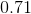7.1%

71%

0.0071%

0.071%

71%

Explanation:

To find the percent, we take the decimal and multiply it by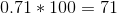The answer is then the equivalent percent.

71%

### Example Question #1 : How To Find Decimal Equivalent To A Percentage

Which of the following numbers is the decimal equivalent of 80%?

0.8

8

80

0.08

0.008

0.8

Explanation:

80% can be thought of as 80% of one. Percents also refer to numbers out of 100, thus, our decimal can be calculated by taking 80/100. This would result in 8/10 after simplifying, which is equivalent to 0.8.

80% = 80/100 = 8/10 = "eight tenths," or 0.8

### Example Question #1 : How To Find Decimal Equivalent To A Percentage

Express 32% in decimal form.Explanation:

To express a percentage as a decimal, imagine a decimal at the end of the percentage. Move the decimal over two places to the left, and you have the decimal expression of a percentage,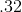### Example Question #2 : How To Find Decimal Equivalent To A Percentage

What is the simplest fraction that represents 88%?Explanation:

The word percent, or per cent, means of every one hundred, so 88% can be expressed: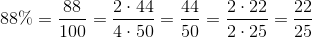### Example Question #3 : How To Find Decimal Equivalent To A Percentage

Andre borrows $80 from you. If he pays you back 40% of that money, how much money is he giving you? Possible Answers:Correct answer:Explanation: To find 40% of$80, you must turn 40% into a decimal.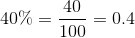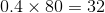### Example Question #1 : How To Find Decimal Equivalent To A Percentage

Convert 74% to a decimal.